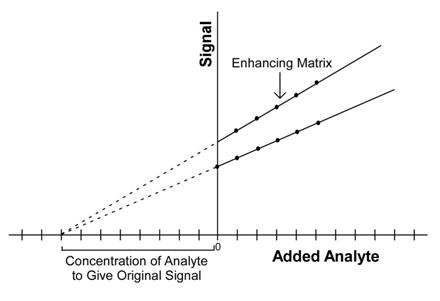# 6.4B: Accounting for Matrix Effects

•• Contributed by Thomas Wenzel
• Professor and Charles A. Dana Professor (Chemistry and Biochemistry) at Bates College

Flame noise, spectral interferences and chemical interferences are all examples of matrix effects. Atomic methods are among the most sensitive of all analysis methods to matrix effects. The previous sections have described ways of trying to account for the possibility of some types of matrix effects. Even with these methods, there is still the possibility that some aspect of the matrix (remember that the matrix is everything except what is being analyzed) either enhances or decreases the signal measured at the detector. A concern is that standard solutions often have a different matrix than the unknowns that are being analyzed.

## Devise a general method that can be used to account for the presence of unknown matrix effects.

A process called standard addition can often be used to assess whether a sample has a matrix effect. If the sample does have a matrix effect, the standard addition procedure will provide a more accurate measurement of the concentration of analyte in the sample than the use of a standard curve. The process involves adding a series of small increments of the analyte to the sample and measuring the signal. The assumption is that the additional analyte experiences the same matrix effects as the species already in the sample. The additional increments are kept small to minimize the chance that they swamp out the matrix and no longer experience the same matrix effects.

The signal for each increment is plotted against the concentration that was added as shown in Figure $$\PageIndex{1}$$. Included in Figure $$\PageIndex{1}$$ are plots for two different samples, both of which have the exact same concentration of analyte. One of the samples has a matrix that enhances the signal relative to the other. An examination of the plots shows that the sample with an enhancing matrix produces a linear plot with a higher slope than the linear plot obtained for the other sample. The plot is then extrapolated back to the X-intercept, which indicates the concentration of analyte that would need to be added to the matrix to obtain the signal measured in the original sample.Figure $$\PageIndex{1}$$: Standard addition plot for two samples with the same concentration. The sample with the higher slope has a matrix that enhances the signal.

The experimental steps involved in conducting a standard addition are more complex than those involving the use of a standard curve. If someone is testing a series of samples with similar properties that have similar matrices, it is desirable to use the standard addition procedure on one or a few samples and compare the concentration to that obtained using a standard curve. If the two results are similar, then it says that the matrix effects are minimal and the use of a standard curve is justified.